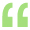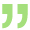# Sfm query

Historically, When the market return changed by 10% the return on the stock of Arihant ltd changed by 16%. If the variance of market is 257.81, then calculate the systematic risk of Arihant Ltd.Minu Sharma (Artcle)     03 May 2016

Beta of Stock = Change in Stock return / Change in Market return

= 16/10 = 1.6

Systematic risk of Stock = Market variance X (Beta Stock)^2

= 257.81 X (1.6)^2

= 660Santosh Mahato (CA student)     03 May 2016

 Originally posted by : Minu SharmaBeta of Stock = Change in Stock return / Change in Market return                        = 16/10 = 1.6 Systematic risk of Stock = Market variance X (Beta Stock)^2                                       = 257.81 X (1.6)^2                                      = 660Agree with MinuMahi Fatima (Student)     04 May 2016

 Originally posted by : Minu SharmaBeta of Stock = Change in Stock return / Change in Market return                        = 16/10 = 1.6 Systematic risk of Stock = Market variance X (Beta Stock)^2                                       = 257.81 X (1.6)^2                                      = 660Great! I am agreed with Minuview more »